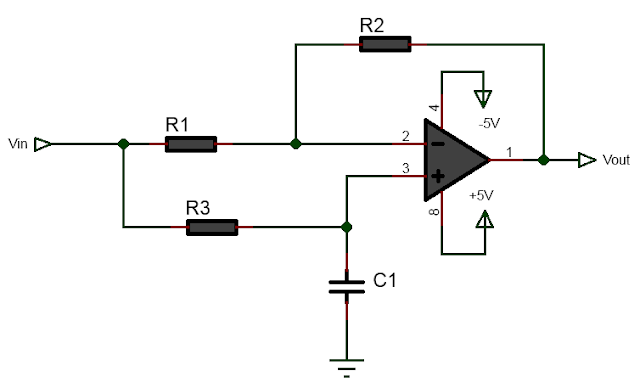# Active Phase Shift Circuit Calculator

Result:Online Phase Shifter Circuit Calculator

A phase shifter circuit is an electronic circuit that is used to shift the phase of a signal without changing its amplitude. It is a fundamental component in many signal processing systems and is used in applications such as audio processing, frequency synthesis, and phase modulation, AM modulation and demodulation, SSB modulation.

Phase Difference Angle

The phase difference angle is given by the following equation,

$$\tan(\frac{\phi}{2})=\frac{f_i}{f_c}$$  -------->(1)

where $$f_c$$ is the cutoff frequency of $$R_3C_1$$ network and $$f_i$$ is the input signal frequency.

Since we have,

$$f_c = \frac{1}{2 \pi R_3 C_1}$$   -------->(2)

Substituting fc in equation(4), we get

$$\tan(\frac{\phi}{2})=2 \pi f_i R_3 C_1$$

Solving for R3

$$R_3=\frac{\tan(\frac{\phi}{2})}{2 \pi f_i C_1}$$   -------->(3)

Using equation(3) the following is calculator that calculates resistor $$R_3$$ from desired phase difference, given capacitor $$C_1$$ and the input frequency.

Example calculation:

Let us suppose that we want to build 90 degree phase shifter circuit. The resistors R1 and R2 can be chosen arbitrarily, say 10KOhm. Let the input frequency be fi=1KHz, then we can use the above equation or the calculator to calculate the required value of resistor R3 given C1. Let C1=0.1uF. Then we have,

$$R_3=\frac{\tan(\frac{\phi}{2})}{2 \pi f_i C_1}$$

or,  $$R_3=\frac{\tan(\frac{90}{2})}{2 \times 3.14 \times 1KHz \times 0.1\mu F} = 1.59k\Omega$$

Following figure shows the 90 degree phase shifter circuit with the calculated values.

Similarly for 180 phase shifter, the value of R3 is 912.35KOhm.

References: